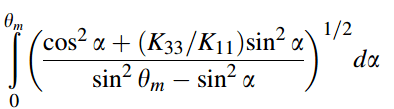# Numerical integration

• MATLAB
Hi all,

i need help solving the following integral using Matlab:* tetam is a parameter and the integration is by alpha.
the answer should be function of tetam
* K33, K11 are constants

Thanks ,

Chen